# December 29, 2016

## Understanding the heart of a Discrete Fourier Transform

### Math

I have been working on understanding the innards of the Discrete Fourier Transform, and I’ve stumbled on what I believe to be the heart of the algorithm. Since then I have been trying to understand why it works. Let’s take a look at this heart: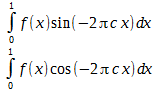These two functions will extract the amplitude and phase of the selected frequency c from the function f. With some experimentation, I have found the following to be true: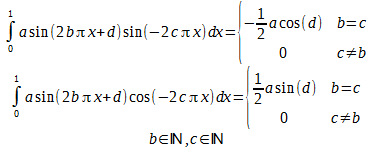What this means is that if we have a sine wave at frequency b, amplitude a and phase d, we can extract phase and amplitude for some test frequency c. Anytime that b and c are equal, we get a non-zero value relating to phase and amplitude. If they are not equal, the answer is simply zero. This is precisely what is needed in a discrete Fourier transform, and why I call it the heart. In a DFT, the function checks the data for each possible integer frequency for the data set, and gets either it’s amplitude and phase, or zero.

First, the discrete Fourier transform in standard notation: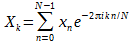Use Euler's formula: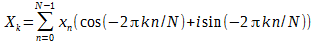Now, assume x is real and break this into the real and imaginary parts and all frequencies: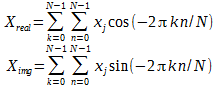Let’s try this on a set of data.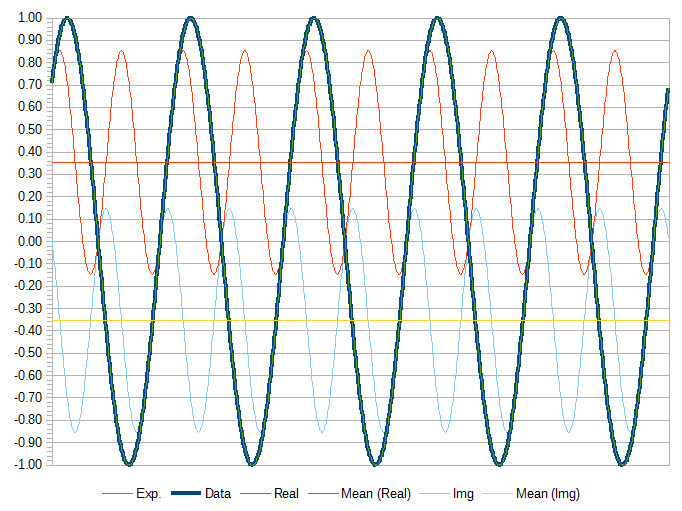Here we have a (b) of frequency of 5 Hz with an amplitude (a) of 1 and phase angle (d) of 45°, and a check frequency (c) of 5 Hz. The sum of the real and imaginary parts is non-zero.

Now let’s try a check frequency of 6 Hz.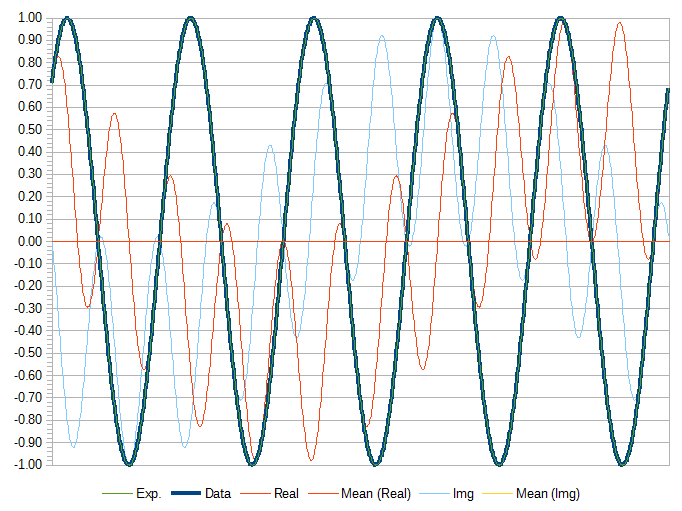Here, the sum of the check frequencies for both real and imaginary are zero. It is more obvious when the check frequency is higher, say 50: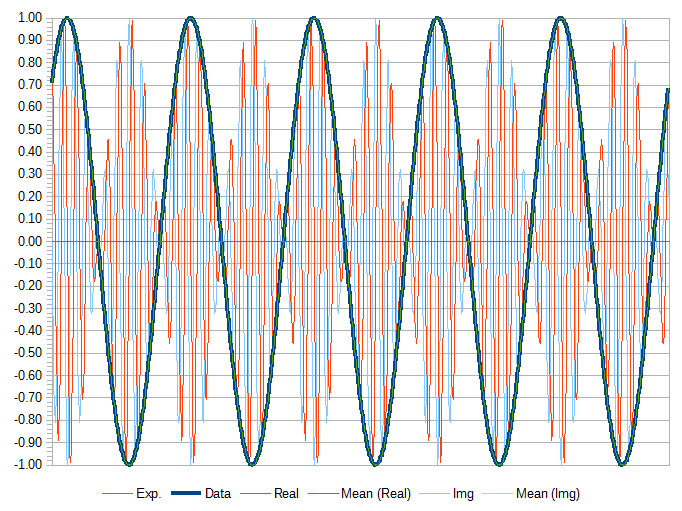Here it is more obvious both the real and imaginary parts spend the same amount of time above and below 0, so overall summing to 0. It also doesn’t matter how many frequencies are in the signal. In the above examples there is just a single frequency, but it will extract amplitude and phase for the check frequency regardless of how many other frequencies are present in the signal.

I haven’t yet figured out why this is the case, but it makes perfect sense why this makes a DFT work.

# December 27, 2016

## LibreOffice Calc Discrete Fourier Transform

### Math, Programming

I've been tired of not having one of these, so today I implemented a macro for doing a Discrete Fourier Transform (DFT) in LibreOffice Calc.  I implemented a DFT rather than an Fast Fourier Transform (FFT) because I wanted to be able to use an arbitrary data size, and because the algorithm is pretty easy to implement.  I cannot remember the last time I did any coding in Basic, but I still don't like it.  I find arrays irritating when implemented with the offset of 1 rather than 0.  Guess I'm too use to C.
' Discrete Fourier Transform (DFT).
' The complexity of this function is O( n^2 ) as it is not a Fast Fourier
' Transform (FFT).  However, unlike as FFT this function works an any input size.
Function dft_guts( inputData As Variant, direction As Double, isScale As boolean )
Dim OFFSET As Integer
OFFSET = LBound( inputData, 1 )

Dim NUMBER As Integer
NUMBER = UBound( inputData, 1 ) - OFFSET

' If we are only given a single column of data, assume that holds the real
' values, and the imaginary values are all 0.  Make a new array with the
' additional imaginary values in it.
If UBound( inputData, 2 ) < 2 Then
Dim newArray( LBound( inputData, 1 ) To UBound( inputData, 1 ), LBound( inputData, 1 ) To UBound( inputData, 1 ) ) As Double

' Copy data into new array.
Dim index As Integer
For index = LBound( inputData, 1 ) To UBound( inputData, 1 )
newArray( index, 1 ) = inputData( index, 1 )
newArray( index, 2 ) = 0.0
Next

' Switch to new array.
inputData = newArray
EndIf

' Storage for results.
Dim outputData( 0 To NUMBER, 1 ) As Double

Dim multiplier As Double
multiplier = direction * 2 * Pi

' If scaled, the scale is the number of samples.
Dim scale As Double
If isScale Then
scale = 1.0 / ( NUMBER + 1 )
Else
scale = 1.0
EndIf

' For each of the sample outputs...
Dim outerIndex As Integer
For outerIndex = 0 To NUMBER

' Start sum at zero.
outputData( outerIndex, 0 ) = 0.0
outputData( outerIndex, 1 ) = 0.0

Dim innerIndex As Integer

' For each input sample...
For innerIndex = 0 To NUMBER

' Angle (radians) at this index.
Dim angle As Double
angle = multiplier * outerIndex * innerIndex / ( NUMBER + 1 )

' Sine and cosine of angle.
Dim cosTerm As Double
Dim sinTerm As Double
cosTerm = cos( angle )
sinTerm = sin( angle )

' Real and imaginary terms at this angle.
Dim realTerm As Double
Dim imaginaryTerm As Double

realTerm = inputData( innerIndex + OFFSET, 1 ) * cosTerm
realTerm = realTerm - inputData( innerIndex + OFFSET, 2 ) * sinTerm

imaginaryTerm = inputData( innerIndex + OFFSET, 1 ) * sinTerm
imaginaryTerm = imaginaryTerm + inputData( innerIndex + OFFSET, 2 ) * cosTerm

' Accumulate results.
outputData( outerIndex, 0 ) = outputData( outerIndex, 0 ) + realTerm
outputData( outerIndex, 1 ) = outputData( outerIndex, 1 ) + imaginaryTerm
Next

' Scale results.
outputData( outerIndex, 0 ) = outputData( outerIndex, 0 ) * scale
outputData( outerIndex, 1 ) = outputData( outerIndex, 1 ) * scale
Next

dft_guts = outputData

End Function

' Discrete Fourier Transform (DFT) in forward direciton (i.e. time->frequency).
Function dft( inputData As Variant )

dft = dft_guts( inputData, -1, false )

End Function

' Inverse Discrete Fourier Transform (iDFT) in reverse direciton (i.e. frequency->time).
Function idft( inputData As Variant )

idft = dft_guts( inputData, 1, true )

End Function
To put this into a Calc document, goto Tool->Macros->Organize Macros->LibreOffice Basic.  Then you can assign the macro to a specific document, or put it in My Macros.  You will probably have to adjust security permissions just to allow the macro to run by going to Tools->Options->Security->Macro Security and setting the level from High (default) to Medium.  If you are not an idiot that shouldn't be a problem.
This implementation isn't fast, but works fine for small data sets of around 50 samples.  Larger sets will still work, but you may be waiting awhile.

# December 10, 2016

## Implementing a Discrete Fourier Transform

### Math, Programming

Sitting fireside at my local coffee shop with a heavy snowstorm taking place outside, listening to Jehan Ariste Alain's Litanies, I came much closer to my goal of understanding how a Fast Fourier Transforms (FFT) works by understanding how to implement a Discrete Fourier Transform (DFT). The equation for a Fourier Transform: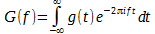Where g( t ) is some function with respect to time, f is some frequency, i is the square root of negative one. You can basically ask the function, what is the phase and amplitude for a given frequency for the time domain function g.

This is fine for transforming functions, but for most programming application we need to transform based on discrete data points. For this we can use a Discrete Fourier Transform (DFT):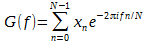Where xn is a data sample at time n, N is the total number of data samples, f is some integer frequency between 0 and N – 1, and G( f ) is amplitude of the given frequency. The discrete form is similar to the general form, except that the function g( t ) is replaced by our discrete sample, and there is now a finite range.

I have seen this equation in many places, but what the exponent is doing isn’t terribly obvious until you remember Euler’s formula: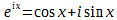Substitute this back in and we get: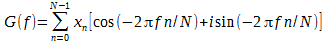Now we just have the imaginary number i as part of the equation, but this is fine because what we actually have is a complex number. They are generally represented in the form: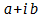And here that breaks down pretty straight-forward: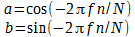In the form I’ve written it, G is a function. But it is actually a set just like x. So a slightly better form maybe: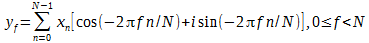There data, x, is actually complex data. Since the discrete data we are putting in consists of real values, it means the imaginary part is just 0. Now we just need to remember how to multiply complex numbers.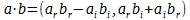Where a and b are complex numbers made for a real part (nr) and an imaginary part (ni) represented n=(nr , ni).

With that, I actually have enough information to implement a Discrete Fourier Transform.

#include <math.h>
#include <stdio.h>

#ifndef M_PI
#define M_PI 3.1415926535897932384626433832795028841971693993751058209749445923
#endif

int main()
{
enum { N = 16 };
double inReal[ N ];
double inImag[ N ];

double outReal[ N ];
double outImag[ N ];

// Generate a signal.
for ( unsigned index = 0; index < N; index += 1 )
{
inReal[ index ]  =       cos( 7 * 2 * M_PI * index / N );
inReal[ index ] += 0.3 * sin( 3 * 2 * M_PI * index / N );
inReal[ index ] += 0.5 * sin( 2 * 2 * M_PI * index / N );
inImag[ index ] = 0;
}

// For each of the sample outputs...
for ( unsigned k = 0; k < N; k += 1 )
{
// Start sum at zero.
outReal[ k ] = 0;
outImag[ k ] = 0;

// For each input sample...
for ( unsigned n = 0; n < N; n += 1 )
{
double cosTerm = cos( -2 * M_PI * k * n / N );
double sinTerm = sin( -2 * M_PI * k * n / N );

outReal[ k ] += inReal[ n ] * cosTerm - inImag[ n ] * sinTerm;
outImag[ k ] += inReal[ n ] * sinTerm + inImag[ n ] * cosTerm;
}
}

// Print the results.
for ( unsigned index = 0; index < N; index += 1 )
printf( "%u: %f %fi\n", index, outReal[ index ] / N, outImag[ index ] / N );

return 0;
}

Here we construct a waveform consisting of 3 sine waves. One at 7 Hz, amplitude of 1, and phase 90°; one at 3 Hz, 0.3 amplitude, and 0°; and 2 Hz, 0.5 amplitude, and 0°. Then the transform, which consists of two loops. The outer loop is for each available integer frequency. The inner loop is the summation above. The last part prints the normalized of the transform, which agree with what I see when I do an FFT on the data.

This is a major step forward in my goal to understand an FFT. I still have a couple of questions, like why the output seems to be split between the upper and lower half. But I have proven (to myself anyway) that I can implement a DFT and that is a lot of progress.

# October 16, 2015

## Ranged Random Numbers with Gaussian distribution

### Math, Programming

I've written in the past about various algorithms I came up with for getting weighted random numbers, but ever since leaning the Box-Muller transform I've been refining my ideas. The Box-Muller transform allows the generation of random numbers with Gaussian distribution. That is, a set of numbers with a known standard deviation. That, in turn, allows for the generation of numbers with some standard statistical properties.

Today I needed a random number between two values, but I wanted something with a known standard deviation. Gaussian distribution does not have a minimum and maximum, theoretically capable of giving any number between negative infinity to positive infinity. However, standard deviation tells us that around 68.2% of the values will be within 1 standard deviation, 95.4% with in 2, and 99.73% with in 3 standard deviations. So by dividing the output of the Box-Muller transform by 3, 99.73% of these values will be between -1 and 1, and only 0.27% will be outside this range. If we ignore values outside this range we can generate a ranged random number generator with a given standard deviation.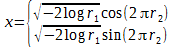There are two inputs, r1 and r2, and two results. The inputs are random values such that 0 ≤ rn ≤ 1. Since there are two input values, there are also two output values. The output is such that -∞ < x < ∞. The Box-Muller transform is kind of the unit circle of Gaussian distribution with 68.2% between -1 and 1, 95.4% between -2 and 2, 99.73% between -3 and 3, and so forth. Multiplying the output of the Box-Muller transform will result in a stream of numbers with a standard deviation of the multiplicand.

Now to get this into a fixed range we just need to scale the results. The problem is that input range is infinity. However, we know that most of the values are closer to the center. So we can simply discard values outside of the desired standard deviation. To illustrate this, let's say we want numbers on average around 75 between 50 and 100 with a standard deviation of 8.

Let xn be the output of the Box-Muller transform. To get a known standard deviation we need to scale down xn. Let s be the scale factor. s= 2d/Δ where d is the desired standard deviation, and Δ=max-min. For our example, d=8, and Δ=(100-50)=50. Thus s = 0.32.

Let y = s xn. Now discard any y where |y| > 1. That is, if the results are larger than one, get a new random number and try again. We now have a value y such that -1 ≤ y ≤ 1, and that we can scale to the desired range. Let z = y Δ/2+ Δ/2+m where m is the minimum value and M is the maximum value. The result z is such that mzM.

Here is a graph of the example: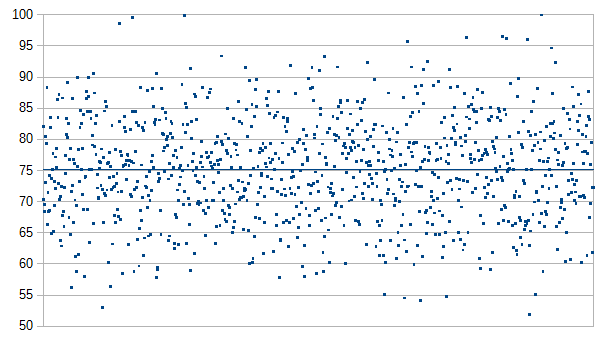This chart shows 1,000 points generated at random with this algorithm. The actual standard deviation is 7.972 which is pretty close to the desired 8.0, average of 75.222, min of 51.950 and max of 99.961. So our algorithm seems to work.

There are some limitations to this system which I haven't yet been able to analyze in depth. This algorithms gets close to the desired standard deviation, but usually comes in under. My guess is that this is due to the amount of data discarded. The larger the standard deviation, the more data is discarded and thus the more this missing data effects the resulting standard deviation. This becomes really noticeable for standard deviation above 18%. For our example range of 50 to 100, 18% is 0.40 * (100-50) = 20. There may be a way to compensate for this by increasing the desired standard deviation to account, but I would have to dive more into the math to come up with a compensation factor.

Here is the C code for the algorithm:

double getGaussianRandom( double standardDeviation )
{
static bool hasRandom = false;
static double randomA = 0;
static double randomB = 0;
static double const TWO_PI = 6.283185307179586476925286766559005768394338502;

double result;

if ( ! hasRandom )
{
randomA = sqrt( -2 * log( getRandom() ) );
randomB = TWO_PI * getRandom();

result = randomA * cos( randomB );
hasRandom = true;
}
else
{
result = randomA * sin( randomB );
hasRandom = false;
}

result *= standardDeviation;

return result;
}

double getRangedGaussianRandom
(
double min,
double max,
double standardDeviation
)
{
double delta = ( max - min ) / 2.0;

double result;
do
{
result = getGaussianRandom( standardDeviation / delta );
}
while ( fabs( result ) > 1.0 );

result *= delta;
result += delta;
result += min;

return result;
}

In this example, the function getRandom returns a number between 0 and 1, but has not been specified.